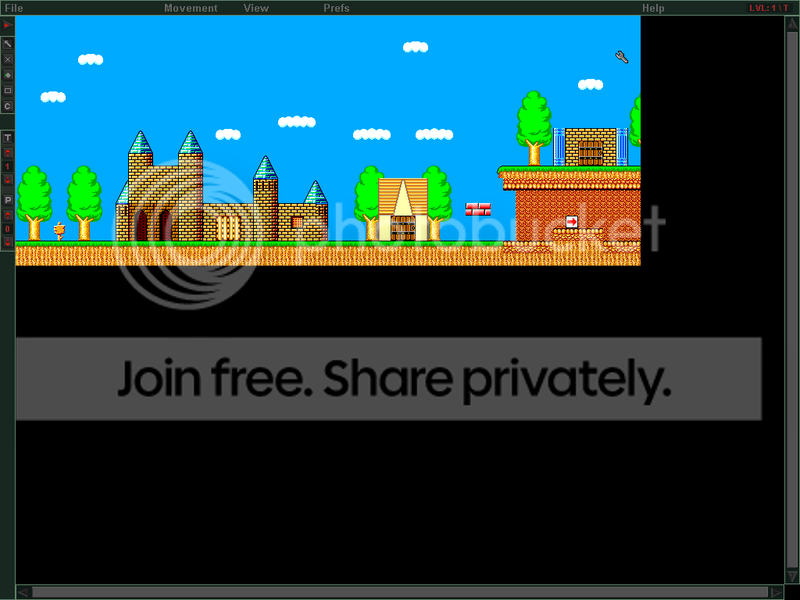-=+=- -=+=- -=+=- -=+=- -=+=- -=+=- -=+=- -=+=- -=+=- -=+=- -=+=- -=+=- -=+=- -=+=- -=+=- -=+=- -=+=- -=+=- -=+=- -=+=- -=+=- -=+=- -=+=- -=+=- -=+=- -=+=- -=+=- -=+=- -=+=- -=+=- (c) WidthPadding Industries 1987 0|81|0 -=+=- -=+=- -=+=- -=+=- -=+=- -=+=- -=+=- -=+=- -=+=- -=+=- -=+=- -=+=- -=+=- -=+=- -=+=- -=+=- -=+=- -=+=- -=+=- -=+=- -=+=- -=+=- -=+=- -=+=- -=+=- -=+=- -=+=- -=+=- -=+=- -=+=-
SoCoder -> Showcase Home -> Apps and Utilities

mike_gCreated : 25 September 2006
Edited : 15 June 2008
Language : Blitz

### Tile Up

2d Tile editor With lots of features

ScreenshotsI wanted a level editor that I could use for more or less any 2d game. This is what I got so far. Has some nice features such as, a config setup that makes it easy to configure any tileset for use within the program. Features random map generation tool. Draw selected areas of tiles. Draw tools like flood fill and rect draw. Saves and loads maps. up to 5 draw levels each with up to 5 placeable levels. Auto generates minimaps for your levels. Nice GUI.

## Latest Update

Very soon I'll put a draw tile function with this to use as an include file in blitzs progs. Then hopefully it should be quicker and easier to make 2d gamesMonday, 25 September 2006, 04:38
JayenkaiI like the Random Generation feature. If you incorporated something like that into an RPG, so that every game was different, then you'd have a pretty fun engine going on.Thursday, 12 April 2007, 14:35
mike_gWell its been almost a year now and I have finally got round to making the two functions to load and draw the maps so you can finally use it to make maps for your Blitz progs. Heres the code:

Its only a quick hash up. I will work on optimizing it. If you use it then you will need to have both the tile and placeable set in the same directory.
Thursday, 12 April 2007, 15:51
Blitz3DmanOnce I learn a bit of C++ maybe I'll make a real nice level editor - that's what I'm going to do once I finish The Running Man, except I'm going to start off in blitz.

By the way, would you mind letting me have a peek at your source?

And I didn't look to see whose showcase this was but the file menu in the screenshot was a dead giveawayThursday, 12 April 2007, 18:24
mike_gErr. I dont know how useful the code would be to you. Theres a lot of it and its a bit of a mess. To start off you might be best off looking at something simple that just shows how to do the basic stuff. Draw tiles, save maps, that sort of stuff. I have an smaller old tile editor that I made ages ago but tbh it sux.
Thursday, 12 April 2007, 18:47
Blitz3DmanNo, not because I don't know how to do it (see my level editor for The Running Man at least I don't think it sucks) but because I want to see how you did it to find out if you are using any better techniques than I so that I can improve my code.
Tuesday, 17 April 2007, 06:36
Blitz3DmanIs there any chance I will get a peek at the code?

If not, okay but I am curious how you did your floodfill and all.
Tuesday, 17 April 2007, 09:37
mike_gNoBut if you want to know how I did the floodfill I used this
algo replacing drawpixel with the tile to draw. Its pretty crude and slow so no good for realtime drawing, but for something like a tile editor its simple and works fine.